# 6. (5 points) Suppose the elementary matrix E is of this form (a) Compute the matrix...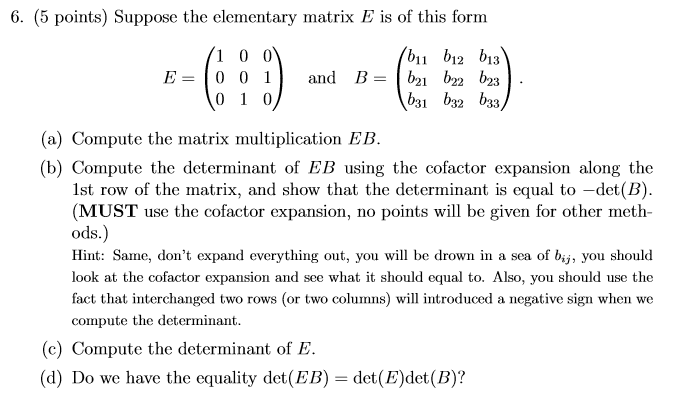6. (5 points) Suppose the elementary matrix E is of this form (a) Compute the matrix multiplication EB (b) Compute the determinant of EB using the cofactor expansion along the 1st row of the matrix, and show that the determinant is equal to -det(B) (MUST use the cofactor expansion, no points will be given for other meth- ods.) Hint: Same, don't expand everything out, you will be drown in a sea of bij, you should look at the cofactor expansion and see what it should equal to. Also, you should use the fact that interchanged two rows (or two columns) will introduced a negative sign when we compute the determinant (c) Compute the determinant of E (d) Do we have the equality det(EB) = det(B)det(B)?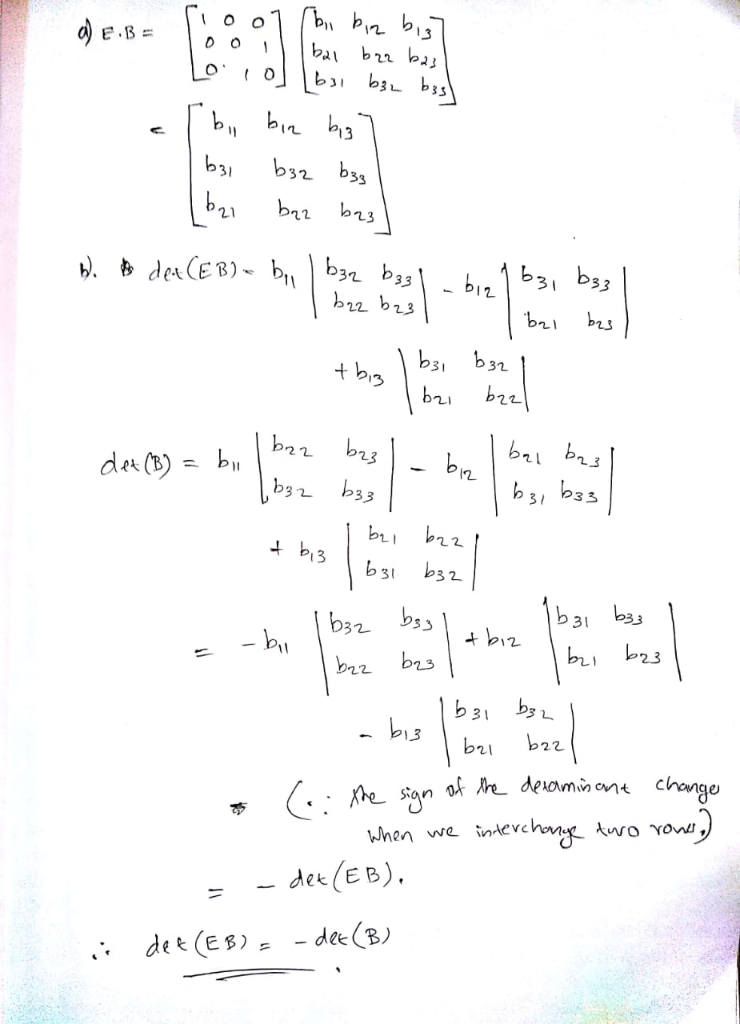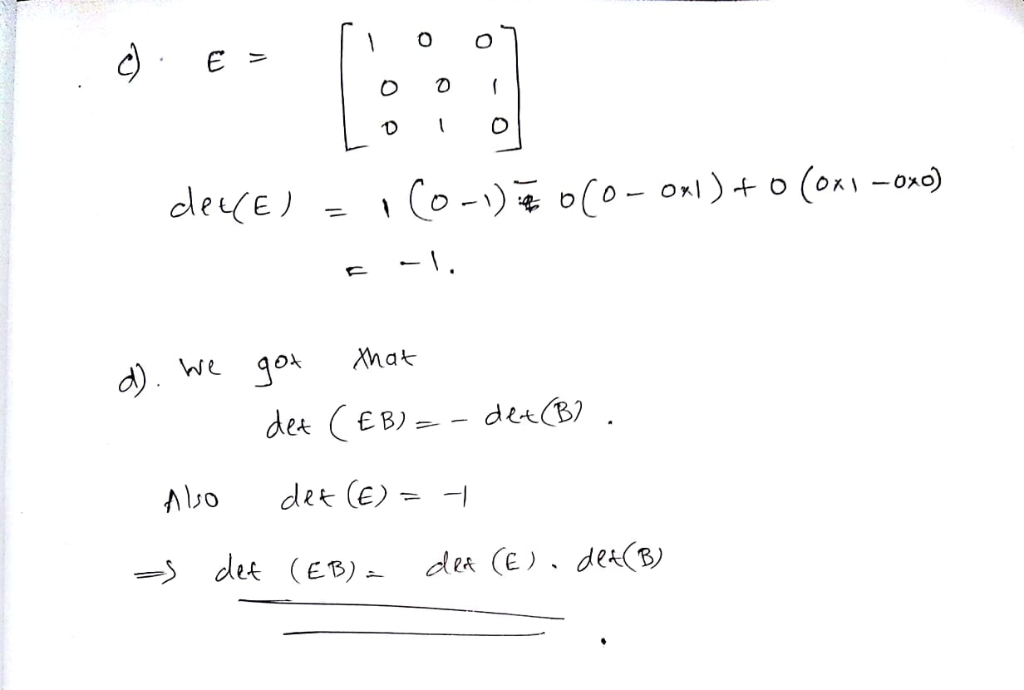#### Earn Coin

Coins can be redeemed for fabulous gifts.

Similar Homework Help Questions
• ### Problem 2. (50 points) Suppose that a 4 x 4 matrix A with rows it. 73,...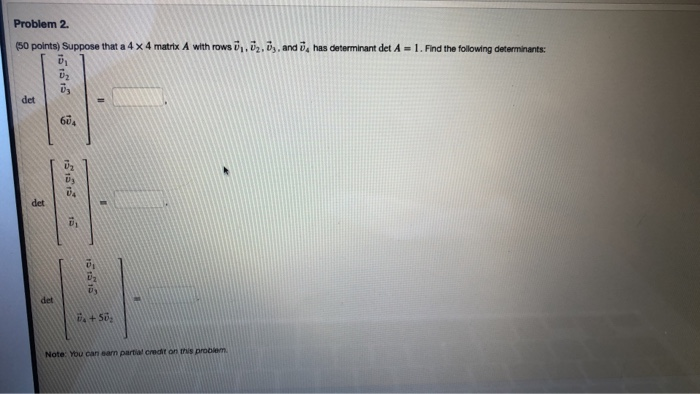Problem 2. (50 points) Suppose that a 4 x 4 matrix A with rows it. 73, 74, and has determinant det A = 1. Find the following determinants: ☺ U2 ü det 604 det det A +50 Note: You can earn partial creat on this problem Problem 1. (50 points) Let Rem -5 -20 -1 -1 - 1 4 16 0 الها (a) Compute dexA Use Cramer's rule to solve the following system 20x -5x -X -4 3 + 16x9...

• ### 6. 10 Suppose a cubic polynomial y2does through the points ( i) fori-1,2,3, 4, where i j for i,j ...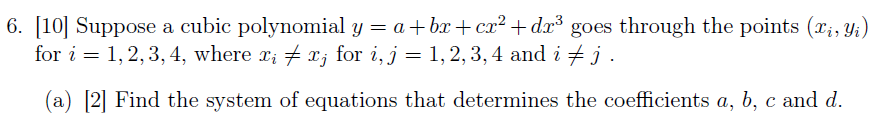6. 10 Suppose a cubic polynomial y2does through the points ( i) fori-1,2,3, 4, where i j for i,j 1,2,3, 4 and i j a) 2 Find the system of equations that determines the coefficients a, b, c and d (b) |6 Find the determinant of the coefficiant matrix using row operations, and show that the coefficient matrix is invertible. Note that you will receive no mark if you compute the determinant using cofactor expansion. (c) [2| Is it possible...

• ### 6. lol suppose a cubic polynomial y = a +br +cr2-dr3 goes through the points (zi, yi) for i 1, 2,...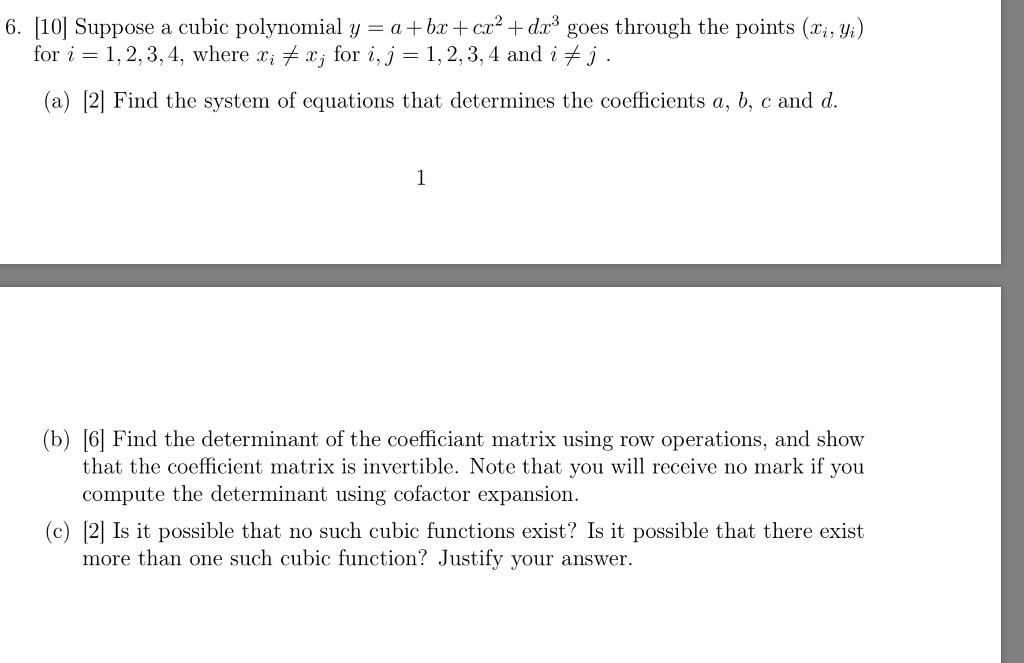6. lol suppose a cubic polynomial y = a +br +cr2-dr3 goes through the points (zi, yi) for i 1, 2,3,4, where r, f a, for i,j 1,2,3,4 and i f j (a) 2 Find the system of equations that determines the coefficients a, b, c and d (b) (61 Find the determinant of the coefficiant matrix using row operations, and show that the coefficient matrix is invertible. Note that you will receive no mark if you compute the determinant...

• ### Answer these explanations: ADDING A MULTIPLE OF THE ith ROW TO THE jth row. 5.4 Example 6: Create a 5 by 5 matrix, E by...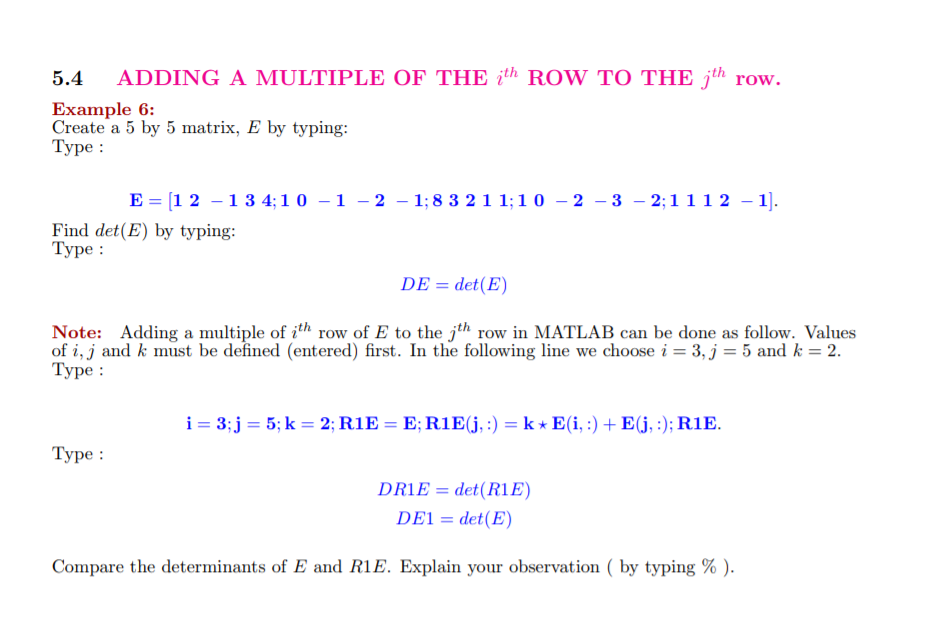Answer these explanations: ADDING A MULTIPLE OF THE ith ROW TO THE jth row. 5.4 Example 6: Create a 5 by 5 matrix, E by typing: Type: ΕΞ11 2-134:10-1-2-1; 8 3 2 11:10-2-3-2:1112-1]. Find det(E) by typing: Type DE det(E) Note: Adding a multiple of ith row of E to the jth row in MATLAB can be done as follow. Values of i, j and k must be defined (entered) first. In the following line we choose i = 3,J...